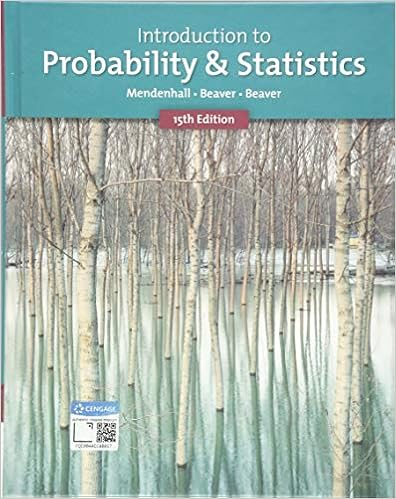# Stats Review Guide 1-5 - CHAPTER ONE Section 2 Data(5 Data...

• Notes
• 20

This preview shows page 1 - 4 out of 20 pages.

##### We have textbook solutions for you!
The document you are viewing contains questions related to this textbook.The document you are viewing contains questions related to this textbook.
Chapter 7 / Exercise 23
Introduction to Probability and Statistics
Beaver/MendenhallExpert Verified
CHAPTER ONESection 2: Data (5) Data:all facts and figured collected, analyzed, and summarized for presentation and interpretationElements:entities on which data are collected (first column) Variable:a characteristic of interest for the elements Nominal scale:Labels or names are used to identify an attribute of an element, numeric codes included as well if they identify a location Ordinal scale:if the data exhibit the properties of nominal data and the order or rank of the data is meaningful. BusinessWeek’s Top 500 companies (ranked) Ratio scale: if the data have all the properties of interval data and the ratio of the two values is meaningful. Distance, height, weight, etc. Zero value exists (means nothing). Qualitative data:include labels or names used to identify an attribute of each element. Nominal or ordinal scale, numeric or nonnumeric. Quantitative data:require numeric values that tell you how much or how many. Interval or ratio scale of measurement. Cross-sectional data:collected at same time Time-series data:collected over several time periods Section 4: Descriptive Statistics (13)Descriptive statistics:Summaries of data presented in a form that is easy for the reader to understand. May be tabular, graphical, or numerical. Section 5: Statistical Inference (15)Population:The set of all elements of interest in a particular study.Sample:A subset of a population. Census:collecting data for an entire populationSample survey:conducting a survey to collect data for a sampleStatistical inference:using data from a sample to make estimates and test hypotheses about the characteristics of a population
##### We have textbook solutions for you!
The document you are viewing contains questions related to this textbook.The document you are viewing contains questions related to this textbook.
Chapter 7 / Exercise 23
Introduction to Probability and Statistics
Beaver/MendenhallExpert Verified
Summary (17)Statistics: the art and science of collecting, analyzing, presenting, and interpreting data CHAPTER TWOSection 1: Summarizing Qualitative Data (28) Frequency Distribution:a tabular summary of data showing the number (frequency) of items in each of several non-overlapping classes – example below Soft DrinkFrequencyCoke19Sprite 17Dr. Pepper 14 Total 50Relative frequency: Frequency of the class / n Percent frequency: relative frequency * 100 Relative frequency distribution: tabular summary of data showing the relative frequency for each classPercent frequency distribution: summarizes the percent frequency of the data for each class Soft DrinkRelative FrequencyPercent FrequencyCoke.38 38 Sprite .34 34Dr. Pepper .2828Total 1100 Bar graph: graphical device for depicting qualitative data summarized in a frequenc distribution. Horizontal axis: categories. Pie chart:another graphical device for depicting qualitative data Section 2: Summarizing Quantitative Data (34)Defining the classes:three steps1. Determine the number of nonoverlapping classes – between 5 and 20 2. Determine the class width (Largest value – smallest value / # classes)3. Determine the class limitsLower class limit:smallest possible data value assigned to that class
Upper class limit: largest Class midpoint:the value halfway between the lower and upper class limits Ogive:a graph of cumulative distribution
•••